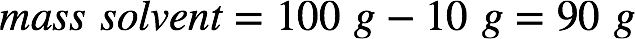# Problem: An aqueous solution is 10.% glucose by mass (d = 1.039 g/mL at 20°C). Calculate its freezing point, boiling point at 1 atm, and osmotic pressure.

###### FREE Expert Solution

The mass percent of a solution is given by:In a 10% by mass glucose (C6H12O6) solution, this means there are 10 g of glucose for every 100 g solution. From this, we can calculate the mass of water (the solvent):We need to find the molality of the solution since we'll use it to calculate the freezing point depression and boiling point elevation. Recall that molality is:The molar mass of glucose is 6(12.01 g/mol C) + 12(1.01 g/mol H) + 6(16.00 g/mol O) = 180.18 g/mol. Calculating the molality of the glucose solution:81% (278 ratings)###### Problem Details

An aqueous solution is 10.% glucose by mass (d = 1.039 g/mL at 20°C). Calculate its freezing point, boiling point at 1 atm, and osmotic pressure.

Frequently Asked Questions

What scientific concept do you need to know in order to solve this problem?

Our tutors have indicated that to solve this problem you will need to apply the Freezing Point Depression concept. If you need more Freezing Point Depression practice, you can also practice Freezing Point Depression practice problems.

What professor is this problem relevant for?

Based on our data, we think this problem is relevant for Professor Evans' class at QC CUNY .

What textbook is this problem found in?

Our data indicates that this problem or a close variation was asked in Chemistry: The Molecular Nature of Matter and Change - Silberberg 8th Edition. You can also practice Chemistry: The Molecular Nature of Matter and Change - Silberberg 8th Edition practice problems.